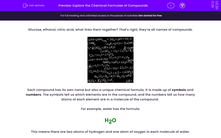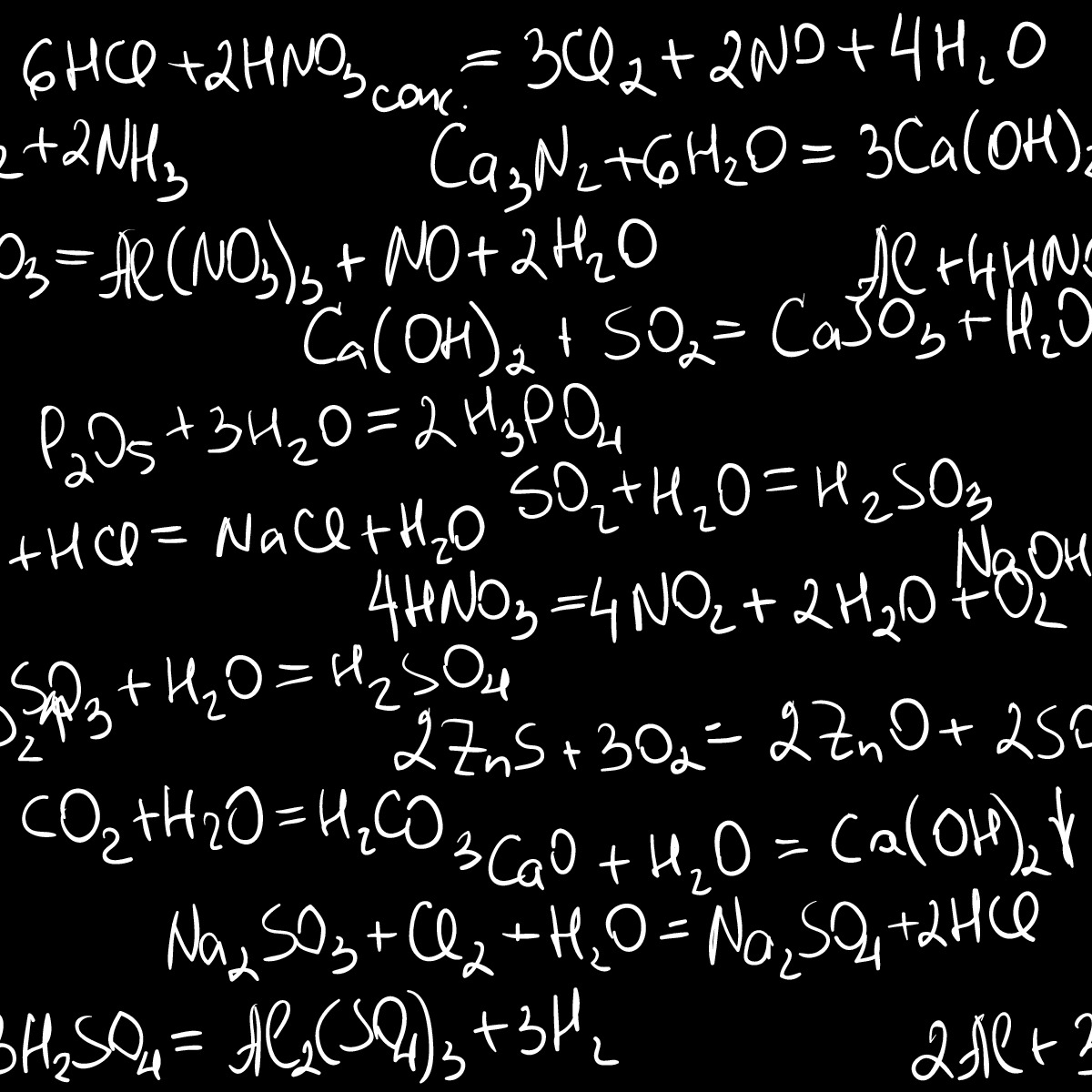# Explore the Chemical Formulae of Compounds

In this worksheet, students will explore the chemical formulae of compounds.Key stage:  KS 3

Curriculum topic:   Chemistry: Atoms, Elements and Compounds

Curriculum subtopic:   Chemical Symbols and Formulae

Difficulty level:#### Worksheet Overview

Glucose, ethanol, citric acid, what links them together? That's right, they're all names of compounds.Each compound has its own name but also a unique chemical formula. It is made up of symbols and numbers. The symbols tell us which elements are in the compound, and the numbers tell us how many atoms of each element are in a molecule of the compound.

For example, water has the formula:

H2O

This means there are two atoms of hydrogen and one atom of oxygen in each molecule of water.

We can apply the same principle to more complex compounds, but don't let the complexity get you confused!

MgSO4 contains three elements, Mg (magnesium), S (sulfur) and O (oxygen). The numbers tell us how many of each atom there are in a molecule of the compound, so in this case, as there is no number after Mg, we can tell there is only one atom present. The same goes for S. But there is a little 4 after O, so that tells us that there are four atoms of oxygen in a molecule of the compound.

By the way, the word 'formulae' is the plural of the word formula. If we're talking about more than one formula, we usually say formulae.Let's have a go at some questions now.

You might find a paper copy of the periodic table helpful for these questions.

### What is EdPlace?

We're your National Curriculum aligned online education content provider helping each child succeed in English, maths and science from year 1 to GCSE. With an EdPlace account you’ll be able to track and measure progress, helping each child achieve their best. We build confidence and attainment by personalising each child’s learning at a level that suits them.

Get started••••